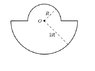# Electric potential in a closed loop wire

cupcake

## Homework Statement

A closed loop of wire that has uniform linear density lambda is bent into the shape shown below, with dimension as indicated. Find the electric potential at point O, assuming it is zero at infinity. (see the attachment)

V = k q /r

## The Attempt at a Solution

Honestly, I am not sure how to attempt this question.
so far, what I did is.. I divided this loop into two part.

for part 1
dV = 2k lambda \int sin theta d(theta), which integral goes from 0 to 90 degree.
and after calculation, it gave me 2klamda with lambda is equal to q/pi*r.

but, I think there's something wrong here.., I don't know what to do..

the correct answer should be 2klambda ( pi + ln 2)

#### Attachments

•cats.jpg
5.7 KB · Views: 461